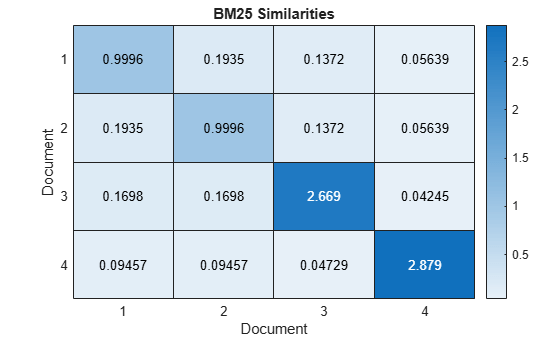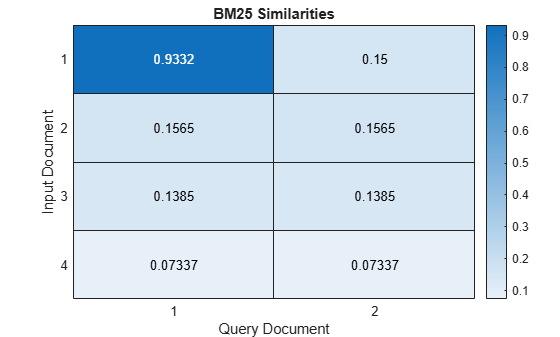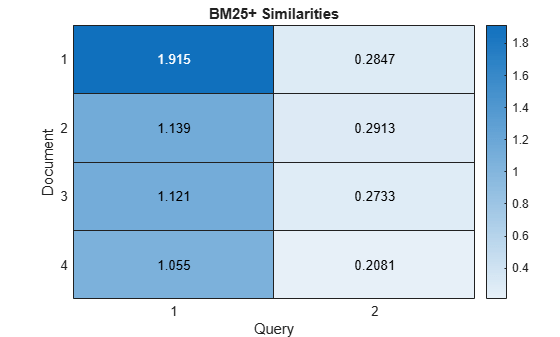# bm25Similarity

Document similarities with BM25 algorithm

## Syntax

``similarities = bm25Similarity(documents)``
``similarities = bm25Similarity(documents,queries)``
``similarities = bm25Similarity(bag)``
``similarities = bm25Similarity(bag,queries)``
``similarities = bm25Similarity(___,Name,Value)``

## Description

Use `bm25Similarity` to calculate document similarities.

By default, this function calculates BM25 similarities. To calculate BM11, BM15, or BM25+ similarities, use the `'DocumentLengthScaling'` and `'DocumentLengthCorrection'` arguments.

example

````similarities = bm25Similarity(documents)` returns the pairwise BM25 similarities between the specified documents. The score in `similarities(i,j)` represents the similarity between `documents(i)` and `documents(j)`.```

example

````similarities = bm25Similarity(documents,queries)` returns similarities between `documents` and `queries`. The score in `similarities(i,j)` represents the similarity between `documents(i)` and `queries(j)`.```

example

````similarities = bm25Similarity(bag)` returns similarities between the documents encoded by the specified bag-of-words or bag-of-n-grams model. The score in `similarities(i,j)` represents the similarity between the `i`th and `j`th documents encoded by `bag`.```
````similarities = bm25Similarity(bag,queries)` returns similarities between the documents encoded by the bag-of-words or bag-of-n-grams model `bag` and the documents specified by `queries`. The score in `similarities(i,j)` represents the similarity between the `i`th document encoded by `bag` and `queries(j)`.```

example

````similarities = bm25Similarity(___,Name,Value)` specifies additional options using one or more name-value pair arguments. For instance, to use the BM25+ algorithm, set the `'DocumentLengthCorrection'` option to a nonzero value.```

## Examples

collapse all

Create an array of tokenized documents.

```textData = [ "the quick brown fox jumped over the lazy dog" "the fast brown fox jumped over the lazy dog" "the lazy dog sat there and did nothing" "the other animals sat there watching"]; documents = tokenizedDocument(textData)```
```documents = 4x1 tokenizedDocument: 9 tokens: the quick brown fox jumped over the lazy dog 9 tokens: the fast brown fox jumped over the lazy dog 8 tokens: the lazy dog sat there and did nothing 6 tokens: the other animals sat there watching ```

Calculate the similarities between them using the `bm25Similarity` function. The output is a sparse matrix.

`similarities = bm25Similarity(documents);`

Visualize the similarities of the documents in a heat map.

```figure heatmap(similarities); xlabel("Document") ylabel("Document") title("BM25 Similarities")```The first three documents have the highest pairwise similarities which indicates that these documents are most similar. The last document has comparatively low pairwise similarities with the other documents which indicates that this document is less like the other documents.

Create an array of input documents.

```str = [ "the quick brown fox jumped over the lazy dog" "the fast fox jumped over the lazy dog" "the dog sat there and did nothing" "the other animals sat there watching"]; documents = tokenizedDocument(str)```
```documents = 4x1 tokenizedDocument: 9 tokens: the quick brown fox jumped over the lazy dog 8 tokens: the fast fox jumped over the lazy dog 7 tokens: the dog sat there and did nothing 6 tokens: the other animals sat there watching ```

Create an array of query documents.

```str = [ "a brown fox leaped over the lazy dog" "another fox leaped over the dog"]; queries = tokenizedDocument(str)```
```queries = 2x1 tokenizedDocument: 8 tokens: a brown fox leaped over the lazy dog 6 tokens: another fox leaped over the dog ```

Calculate the similarities between input documents and query documents using the `bm25Similarity` function. The output is a sparse matrix. The score in `similarities(i,j)` represents the similarity between `documents(i)` and `queries(j)`.

`similarities = bm25Similarity(documents,queries);`

Visualize the similarities of the documents in a heat map.

```figure heatmap(similarities); xlabel("Query Document") ylabel("Input Document") title("BM25 Similarities")```In this case, the first input document is most like the first query document.

Create a bag-of-words model from the text data in `sonnets.csv`.

```filename = "sonnets.csv"; tbl = readtable(filename,'TextType','string'); textData = tbl.Sonnet; documents = tokenizedDocument(textData); bag = bagOfWords(documents)```
```bag = bagOfWords with properties: Counts: [154x3527 double] Vocabulary: [1x3527 string] NumWords: 3527 NumDocuments: 154 ```

Calculate similarities between the sonnets using the `bm25Similarity` function. The output is a sparse matrix.

`similarities = bm25Similarity(bag);`

Visualize the similarities between the first five documents in a heat map.

```figure heatmap(similarities(1:5,1:5)); xlabel("Document") ylabel("Document") title("BM25 Similarities")```The BM25+ algorithm addresses a limitation of the BM25 algorithm: the component of the term-frequency normalization by document length is not properly lower bounded. As a result of this limitation, long documents which do not match the query term can often be scored unfairly by BM25 as having a similar relevance to shorter documents that do not contain the query term.

BM25+ addresses this limitation by using a document length correction factor (the value of the `'DocumentLengthScaling'` name-value pair). This factor prevents the algorithm from over-penalizing long documents.

Create two arrays of tokenized documents.

```textData1 = [ "the quick brown fox jumped over the lazy dog" "the fast fox jumped over the lazy dog" "the dog sat there and did nothing" "the other animals sat there watching"]; documents1 = tokenizedDocument(textData1)```
```documents1 = 4x1 tokenizedDocument: 9 tokens: the quick brown fox jumped over the lazy dog 8 tokens: the fast fox jumped over the lazy dog 7 tokens: the dog sat there and did nothing 6 tokens: the other animals sat there watching ```
```textData2 = [ "a brown fox leaped over the lazy dog" "another fox leaped over the dog"]; documents2 = tokenizedDocument(textData2)```
```documents2 = 2x1 tokenizedDocument: 8 tokens: a brown fox leaped over the lazy dog 6 tokens: another fox leaped over the dog ```

To calculate the BM25+ document similarities, use the `bm25Similarity` function and set the `'DocumentLengthCorrection'` option to a nonzero value. In this case, set the `'DocumentLengthCorrection'` option to 1.

`similarities = bm25Similarity(documents1,documents2,'DocumentLengthCorrection',1);`

Visualize the similarities of the documents in a heat map.

```figure heatmap(similarities); xlabel("Query") ylabel("Document") title("BM25+ Similarities")```Here, when compared with the example Similarity Between Documents, the scores show more similarity between the input documents and the first query document.

## Input Arguments

collapse all

Input documents, specified as a `tokenizedDocument` array, a string array of words, or a cell array of character vectors. If `documents` is not a `tokenizedDocument` array, then it must be a row vector representing a single document, where each element is a word. To specify multiple documents, use a `tokenizedDocument` array.

Input bag-of-words or bag-of-n-grams model, specified as a `bagOfWords` object or a `bagOfNgrams` object. If `bag` is a `bagOfNgrams` object, then the function treats each n-gram as a single word.

Set of query documents, specified as one of the following:

• A `tokenizedDocument` array

• A `bagOfWords` or `bagOfNgrams` object

• A 1-by-N string array representing a single document, where each element is a word

• A 1-by-N cell array of character vectors representing a single document, where each element is a word

To compute term frequency and inverse document frequency statistics, the function encodes `queries` using a bag-of-words model. The model it uses depends on the syntax you call it with. If your syntax specifies the input argument `documents`, then it uses `bagOfWords(documents)`. If your syntax specifies `bag`, then it uses `bag`.

### Name-Value Pair Arguments

Specify optional comma-separated pairs of `Name,Value` arguments. `Name` is the argument name and `Value` is the corresponding value. `Name` must appear inside quotes. You can specify several name and value pair arguments in any order as `Name1,Value1,...,NameN,ValueN`.

Example: `bm25Similarity(documents,'TFScaling',1.5)` returns the pairwise similarities for the specified documents and sets the token frequency scaling factor to 1.5.

Method to compute inverse document frequency factor, specified as the comma-separated pair consisting of `'IDFWeight'` and one of the following:

• `'textrank'` – Use TextRank IDF weighting . For each term, set the IDF factor to

• `log((N-NT+0.5)/(NT+0.5))` if the term occurs in more than half of the documents, where `N` is the number of documents in the input data and `NT` is the number of documents in the input data containing each term.

• `IDFCorrection*avgIDF` if the term occurs in half of the documents or f, where `avgIDF` is the average IDF of all tokens.

• `'classic-bm25'` – For each term, set the IDF factor to `log((N-NT+0.5)/(NT+0.5))`.

• `'normal'` – For each term, set the IDF factor to `log(N/NT)`.

• `'unary'` – For each term, set the IDF factor to 1.

• `'smooth'` – For each term, set the IDF factor to `log(1+NT/NT)`.

• `'max'` – For each term, set the IDF factor to `log(1+max(NT)/NT)`.

• `'probabilistic'` – For each term, set the IDF factor to `log((N-NT)/NT)`.

where `N` is the number of documents in the input data and `NT` is the number of documents in the input data containing each term.

Term frequency scaling factor, specified as the comma-separated pair consisting of `'TFScaling'` and a nonnegative scalar.

This option corresponds to the value k in the BM25 algorithm. For more information, see BM25.

Data Types: `single` | `double` | `int8` | `int16` | `int32` | `int64` | `uint8` | `uint16` | `uint32` | `uint64`

Document length scaling factor, specified as the comma-separated pair consisting of `'DocumentLengthScaling'` and a scalar in the range [0,1].

This option corresponds to the value b in the BM25 algorithm. When b=1, the BM25 algorithm is equivalent to BM11. When b=0, the BM25 algorithm is equivalent to BM15. For more information, see BM11, BM15, or BM25.

Data Types: `double`

Inverse document frequency correction factor, specified as the comma-separated pair consisting of `'IDFCorrection'` and a nonnegative scalar.

This option only applies when `'IDFWeight'` is `'textrank'`.

Data Types: `single` | `double` | `int8` | `int16` | `int32` | `int64` | `uint8` | `uint16` | `uint32` | `uint64`

Document length correction factor, specified as the comma-separated pair consisting of `'DocumentLengthCorrection'` and a nonnegative scalar.

This option corresponds to the value $\delta$ in the BM25+ algorithm. If the document length correction factor is nonzero, then the `bm25Similarity` function uses the BM25+ algorithm. Otherwise, the function uses the BM25 algorithm. For more information, see BM25+.

Data Types: `double`

## Output Arguments

collapse all

BM25 similarity scores, returned as a sparse matrix:

• Given a single array of tokenized documents, `similarities` is a N-by-N nonsymmetric matrix, where `similarities(i,j)` represents the similarity between `documents(i)` and `documents(j)`, and N is the number of input documents.

• Given an array of tokenized documents and a set of query documents, `similarities` is an N1-by-N2 matrix, where `similarities(i,j)` represents the similarity between `documents(i)` and the `j`th query document, and N1 and N2 represents the number of documents in `documents` and `queries`, respectively.

• Given a single bag-of-words or bag-of-n-grams model, `similarities` is a `bag.NumDocuments`-by-`bag.NumDocuments` nonsymmetric matrix, where `similarities(i,j)` represents the similarity between the `i`th and `j`th documents encoded by `bag`.

• Given a bag-of-words or bag-of-n-grams models and a set of query documents, `similarities` is a `bag.NumDocuments`-by-N2 matrix, where `similarities(i,j)` represents the similarity between the `i`th document encoded by `bag` and the `j`th document in `queries`, and N2 corresponds to the number of documents in `queries`.

## Tips

• The BM25 algorithm aggregates and uses information from all the documents in the input data via the term frequency (TF) and inverse document frequency (IDF) based options. This behavior means that the same pair of documents can yield different BM25 similarity scores when the function is given different collections of documents.

• The BM25 algorithm can output different scores when comparing documents to themselves. This behavior is due to the use of the IDF weights and the document length in the BM25 algorithm.

## Algorithms

collapse all

### BM25

Given a document from a collection of documents $\mathcal{D}$, and a query document, the BM25 score is given by

where

• Count(word,document) denotes the frequency of word in document.

• $\overline{n}$ denotes the average document length in $\mathcal{D}$.

• k denotes the term frequency scaling factor (the value of the `'TFScaling'` name-value pair argument). This factor dampens the influence of frequently appearing terms on the BM25 score.

• b denotes the document length scaling factor (the value of the `'DocumentLengthScaling'` name-value pair argument). This factor controls how the length of a document influences the BM25 score. When b=1, the BM25 algorithm is equivalent to BM11. When b=0, the BM25 algorithm is equivalent to BM15.

• $\text{IDF}\left(\text{word},\mathcal{D}\right)$ is the inverse document frequency of the specified word given the collection of documents $\mathcal{D}$.

### BM25+

The BM25+ algorithm addresses a limitation of the BM25 algorithm: the component of the term-frequency normalization by document length is not properly lower bounded. As a result of this limitation, long documents which do not match the query term can often be scored unfairly by BM25 as having a similar relevance to shorter documents that do not contain the query term.

The BM25+ algorithm is the same as the BM25 algorithm with one extra parameter. Given a document from a collection of documents $\mathcal{D}$ and a query document, the BM25+ score is given by

where the extra parameter $\delta$ denotes the document length correction factor (the value of the `'DocumentLengthScaling'` name-value pair). This factor prevents the algorithm from over-penalizing long documents.

### BM11

BM11 is a special case of BM25 when b=1.

Given a document from a collection of documents $\mathcal{D}$, and a query document, the BM11 score is given by

### BM15

BM15 is a special case of BM25 when b=0.

Given a document from a collection of documents $\mathcal{D}$, and a query document, the BM15 score is given by

 Robertson, Stephen, and Hugo Zaragoza. "The Probabilistic Relevance Framework: BM25 and Beyond." Foundations and Trends® in Information Retrieval 3, no. 4 (2009): 333-389.

 Barrios, Federico, Federico López, Luis Argerich, and Rosa Wachenchauzer. "Variations of the Similarity Function of TextRank for Automated Summarization." arXiv preprint arXiv:1602.03606 (2016).